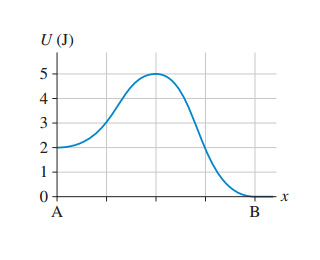# Problem: A particle in has a mass of 100 g.What minimum speed does the particle need at point A to reach point B?What minimum speed does the particle need at point B to reach point A?

⚠️Our tutors found the solution shown to be helpful for the problem you're searching for. We don't have the exact solution yet.

###### Problem Details

A particle inhas a mass of 100 g.

What minimum speed does the particle need at point A to reach point B?

What minimum speed does the particle need at point B to reach point A?